# Insight into Photoshop 7.0 Blending Modes

I constructed this page to satisfy my own curiosity about the functioning of Photoshops Blend modes. Some Blend modes may be intuitive, but many are certainly very obscure as far as what exactly they are doing to your color information.

By using a controlled set of two greyscale images, I have attempted to demonstrate here how each Blend mode works. As a developer, my analysis might seem a little abstract and mathematical but I have accompanied all of my examples with diagrams to demonstrate precisely what each mode does.

I started with a simple gradient fill from black to white and another similar image rotated 90°. These are the two images (A and B) that are used for all of the following Blends. Since the eye can be easily fooled by gradual gradients, I have added rollovers to the Blends on this page to help discern where the exact contours are.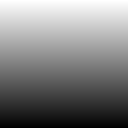image A image B

When Photoshop applies the Blends to full color images it does so on each of three channels; red, green and blue (for RGB) or four channels: cyan, magenta, yellow and black (for CMYK). Each channel is Blended independently as shown here and what you see is the combined effect for all channels. All Blends were done in indexed RGB color mode in Photoshop 7.0.

Although many of the Blends are commutative, some are not. By commutative I simply mean that the layers can be swapped and will see the same result. Many of the Blends are not commutative, such as Color Burn. That is, they depend on which way around the layers are. This was a surprise to myself, as I had previously thought that ALL of the Blend modes were commutative.

I do not here investigate the four color-Blending modes at the end of Photoshop's list; hue, saturation, color, and luminosity. They have some significantly different properties that are best left for another discussion.

## Blend Modes:

Mouse over any blend to enhance contours.

For all math involved here: black (#00) = 0, mid-grey (#80) = ½, white (#ff) = 1.
Division in color math is "clamped" to the range of values between 0 and 1. In other words, results less than zero become zero and results larger than one become one.

Notice that in all of the blends in the first three groups, all resulting images have black in the lower left, and white in the upper right. They are black where both Base and Blend are black and they are white where both Base and Blend are white. They all serve to alter the contrast in between. The fourth group however, involves an inversion (or a negation) when either Base or Blend are white.

The first group of blends generally tend to darken the image. Three out of four of them are commutative. Group one blends are generally inversed versions of the group two blends.

Darken:
commutative

R = min(Base,Blend)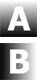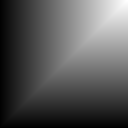Multiply:
commutative

R = Base × Blend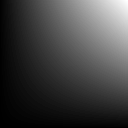Color Burn:
non-commutative

R = 1 - (1-Base) / BlendLinear Burn:
commutative

R = Base + Blend - 1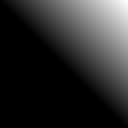The second group of blends generally tend to lighten the image. Three out of four of them are commutative. Group two blends are generally inversed versions of the group one blends.

Lighten:
commutative

R = max(Base,Blend)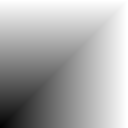Screen:
commutative

R = 1 - (1-Base) × (1-Blend)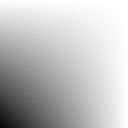Color Dodge:
non-commutative

R = Base / (1-Blend)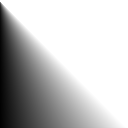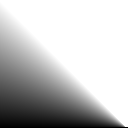Linear Dodge:
commutative

R = Base + Blend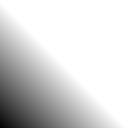The third group of Blends are combinations of the first two groups. None of them are commutative.

Overlay:
non-commutative
a combination of multiply and screen
also the same as Hard Light commuted
if (Base > ½) R = 1 - (1-2×(Base-½)) × (1-Blend)
if (Base <= ½) R = (2×Base) × Blend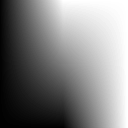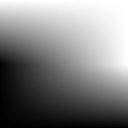Soft Light:
non-commutative
a combination of multiply and screen
(Soft Light formula is only approximate)
if (Blend > ½) R = 1 - (1-Base) × (1-(Blend-½))
if (Blend <= ½) R = Base × (Blend+½)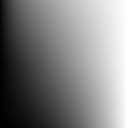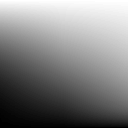Hard Light:
non-commutative
a combination of multiply and screen
also the same as Overlay commuted
if (Blend > ½) R = 1 - (1-Base) × (1-2×(Blend-½))
if (Blend <= ½) R = Base × (2×Blend)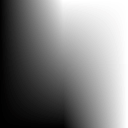Vivid Light:
non-commutative
a combination of color burn and color dodge
if (Blend > ½) R = 1 - (1-Base) / (2×(Blend-½))
if (Blend <= ½) R = Base / (1-2×Blend)Linear Light:
non-commutative
a combination of linear burn and linear dodge
if (Blend > ½) R = Base + 2×(Blend-½)
if (Blend <= ½) R = Base + 2×Blend - 1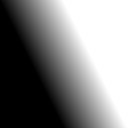Pin Light:
non-commutative
a combination of darken and lighten
if (Blend > ½) R = max(Base,2×(Blend-½))
if (Blend <= ½) R = min(Base,2×Blend))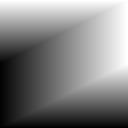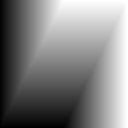The fourth group of blends involve varying degrees of negation in one image depending on values in the other. Both of them are commutative.

Difference:
commutative

R = | Base - Blend |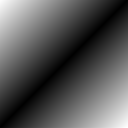Exclusion:
commutative

R = ½ - 2×(Base-½)×(Blend-½)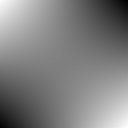questions & comments: yet another web page Dunn by Paul 11/11/2006 © 2006